## 机器学习的基本概念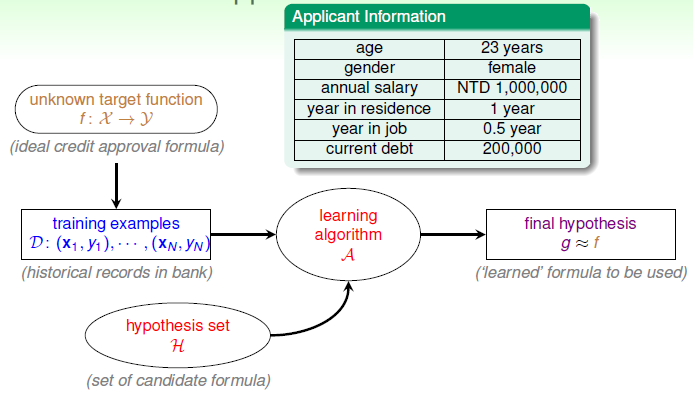## 感知机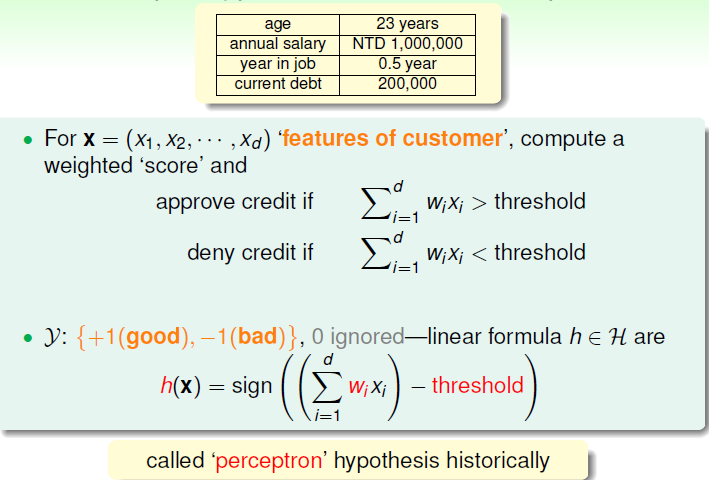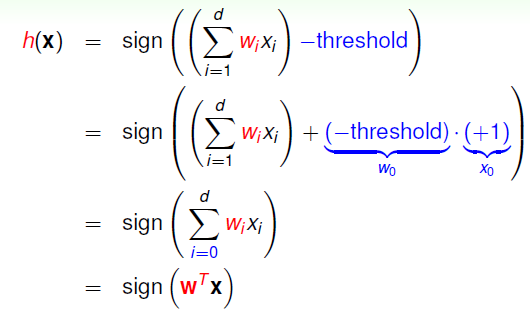### PLA

1）随机初始化参数 $w$
2）利用参数 $w$ 预测每个样本点的值并与其实际的值比较，对于分类错误的样本点$(x_n, y_n)$，利用公式 $w = w + y_nx_n$ 更新参数 $w$ 的值
3）重复上面的过程直到所有的样本点都能够被参数 $w$ 正确预测。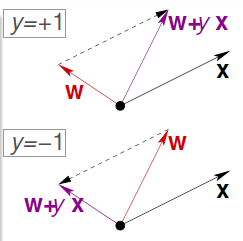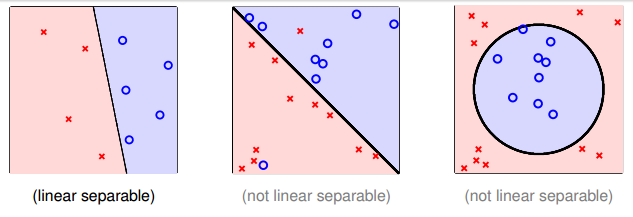### 收敛性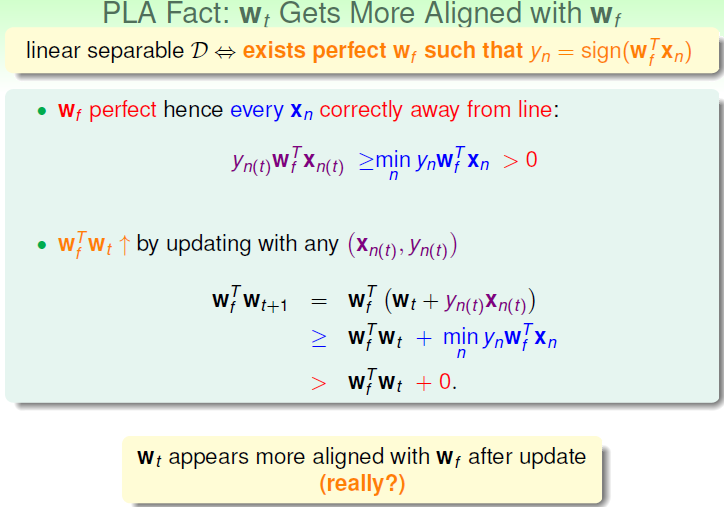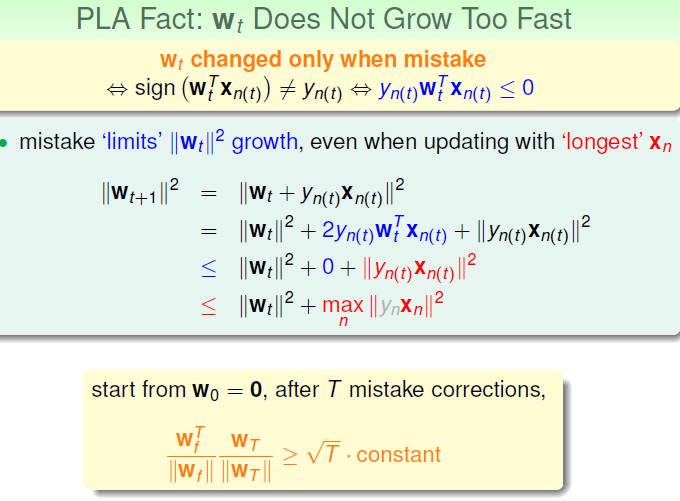$$w_f^Tw_T \ge w_f^Tw_{T-1} + \min_n y_nw_f^Tx_n \ge T\min_n y_nw_f^Tx_n$$

$$||w_T||^2 \le ||w_{T-1}||^2 + \max_n||x_n||^2 \le T\max_n||x_n||^2\ ||w_T|| \le \sqrt{T} \max_n||x_n||$$

$$\frac{w_f^T}{||w_f^T||}\frac{w_T}{||w_T||} \ge \frac{T\min_n y_n^Tw_f^Tx_n}{||w_f^T||\sqrt{T} \max_n||x_n||} = \sqrt{T} \frac{\min_n y_n\frac{w_f^T}{||w_f^T||}x_n}{\max_n||x_n||} = \sqrt{T} * constant$$

$$\max_n||x||^2 = R^2, \rho = \min_n y_n\frac{w_f^T}{||w_f^T||}x_n$$

$$T \le \frac{R^2}{\rho^2}$$

### 优缺点及改进

PLA 的优点和缺点都非常明显，其中优点是简单，易于实现，但是缺点是假设了数据是线性可分的，然而事先并无法知道数据是否线性可分的。正如上面提到的一样，假如将PLA 用在线性不可分的数据中时，会导致PLA永远都无法对样本进行正确分类从而陷入到死循环中。